# TUTORIAL ON SUPERCUBE SOLVING (4) (Some Additional Tricks)

In the previous two tutorials, I have shown the basic procedures of solving odd- and even-order Supercubes. In this tutorial, I will provide some additional tricks. These tricks together with the basic procedures are most probably not the quickest ways of solving Supercubes. But learning them can help you understand more about Supercubes. In what follows, I will from time to time refer to the various steps and sub-steps of solving Supercubes, which I assume that the readers are familiar with by now. If necessary, please refer to the previous tutorials.

## Positions and Orientations of Core Center Pieces of Odd-order Supercubes

When doing Steps I to III to match the center pieces of odd-order Supercubes, a natural way is to move all non-core center pieces of a particular color to the appropriate positions on the face where the core center piece of the same color is located, and orient these pieces to match the orientation of the core center piece. What would happen if you wrongly place or orient some of the core center pieces (intentionally or unintentionally)? I first discuss the situation that some core center pieces are wrongly placed. Note that it is absolutely possible to restore the non-core center pieces to the wrong faces, as illustrated by the following diagram:The diagram above shows two views of a 7th-order Supercube in which the positions of the red and yellow core center pices are interchanged with each other, while all other center pieces are in the correct positions. This situation can arise only when the edge pieces and corner pieces of the Supercube are not yet solved. When proceeding to the subsequent steps, the situation shown above cannot be maintained because it does not match the original structure of the Supercube. For example, the situation above requires a "yellow-blue-red" corner piece where the yellow, blue and red facelets are facing forward, upward and rightward, respectively. But such a corner piece simply does not exist.

However, some situations can persist until the last step of solving Supercubes. The diagram below shows one possible situation:If you encounter a situation like the one shown above, do not restart from Step I. You only need to make use of the following formulas:

 (f, u, r)(b, d, l) Formula: RmFm−1Rm−1Fm (1) (f, u, l)(b, d, r) Formula: RmFmRm−1Fm−1 (2) (f, d, r)(b, u, l) Formula: Rm−1FmRmFm−1 (3) (f, d, l)(b, u, r) Formula: Rm−1Fm−1RmFm (4) (f, r, u)(b, l, d) Formula: Fm−1RmFmRm−1 (5) (f, l, u)(b, r, d) Formula: FmRmFm−1Rm−1 (6) (f, r, d)(b, l, u) Formula: FmRm−1Fm−1Rm (7) (f, l, d)(b, r, u) Formula: Fm−1Rm−1FmRm (8) (r, l)(u, d) Formula: (1) • (6) (9) (f, b)(u, d) Formula: (1) • (7) (10) (f, b)(r, l) Formula: (1) • (8) (11)

The names of these formulas are given in "cycle notation" which tells you how the core center pieces will move by virtue of these formulas. For example, in (f, u, r)(b, d, l) the first bracket means moving the front core center piece to the location of the top core center piece, which is moved to the location of the right core center piece, which is moved to the location of the front core center piece, hence forming a cycle. The second bracket means another cycle. Note that each of formulas (9) - (11) is the composition of two formulas from (1) - (8). For example, (1) • (6) means the composition of (1) and (6), i.e. doing (1) first and then (6).

I next discuss the situation that some core center pieces are wrongly oriented. Again, some situations of wrongly oriented core center pieces can arise only when the edge pieces and corner pieces of the Supercube are not yet solved. But some situations can persist until the last step of solving Supercubes. The diagram below shows one possible situation: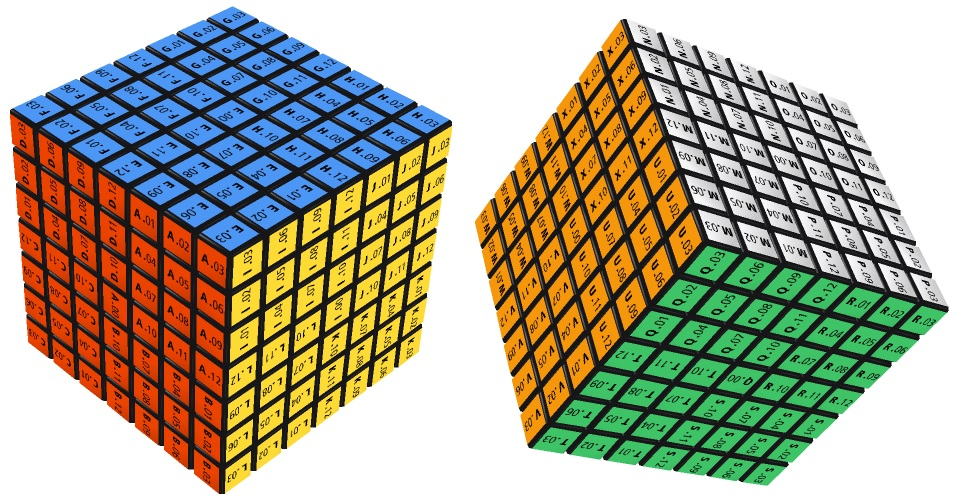In the diagram above, apart from the red and green core center pieces which are each wrongly oriented by 90°, all other core center pieces are oriented correctly. Again, if you encounter a situation like this one, do not restart from Step I because you can fix it by making use of the following formulas:

 Two Center Rotation Formula: L−1 R F−1 B U−1 D Ln D−1 U B−1 F R−1 L U−n (12) One Center Rotation Formula: (U R L U2 R−1 L−1)2 (13)

Recall that these two formulas can be used to rotate the centers of a Supercube. Here "center" means all center pieces on a particular face, not just the core center piece. The two formulas above tell you to rotate the outer surfaces of a Supercube. But if you rotate the outer surfaces together with some of the non-core inner layers, the net effect will be different. For example, for a 7th-order Supercube, if you use Formula (13) to rotate the outer surface together with the two non-core inner layers closest to the outer surface, then the net effect is rotation of just the core center piece on the top face. This is equivalent to using the following formula:

(UU1U2 RR1R2 LL1L2 U2U12U22 R−1R1−1R2−1 L−1L1−1L2−1)2     (14)

The following diagram shows the effect of applying (14) to a solved 7th-order Supercube (with the red face facing forward and the blue face facing upward):Note that in the diagram above, only the top core center piece (i.e. the "E.00" piece) has been rotated by 180° while all other pieces remain unchanged.

## OLL Parity Errors of Even-order Supercubes

In TUTORIAL ON SUPERCUBE SOLVING (3) (Solving Even-Order Supercubes), I provided four swapping / flipping formulas which are reproduced below:

One Side Swapping Formula: Two Side Swapping Formula: Lx−1 U2 Lx−1 U2 F2 Lx−1 F2 Rx U2 Rx−1 U2 Lx2 (15) F2 Lx−1 F B−1 R2 F−1 B Rx Lx F B−1 R2 F−1 B Rx−1 F2 (16) Rx2 B2 U2 Lx U2 Rx−1 U2 Rx U2 F2 Rx F2 Lx−1 B2 Rx2 (17) U−1 F R−1 U F−1 Rx−1 Lx F U−1 R F−1 U Rx Lx−1 (18)

You will recall that these swapping / flipping formulas have to be used in several steps / sub-steps of solving even-order Supercubes, namely Sub-step 2 of Step III, Step V, and Sub-steps 4 and 6 of Step VI. Since these formulas are long and not easy to remember, it would be most undesirable if you have to apply them time and again. While I do not know how to keep the use of these formulas to the minimum, I now provide some tricks which can reduce their use. These formulas are related to two types of errors: OLL parity errors and PLL parity errors. The OLL parity errors are illustrated by the following diagram: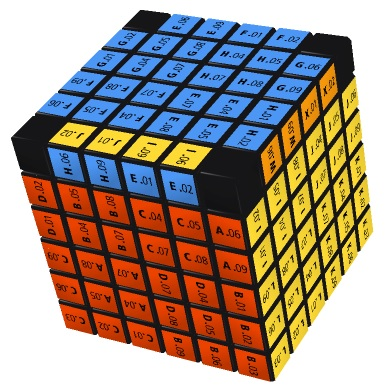The diagram above shows a 6th order Supercube at the moment immediately before Sub-step 4 of Step VI. Among the 4 edges on the top face of this Supercube, only one edge (namely, the "yellow-blue" edge) is oriented incorrectly with its blue facelet not facing upward. Generally speaking, we say that an OLL parity error occurs whenever there is 1 or 3 top edges oriented incorrectly at the moment immediately before Sub-step 4. Note that this type of errors will only arise in even-order Supercubes.

To avoid OLL parity errors, you have to turn back to Sub-step 2 of Step III. Recall that the objective of this sub-step is to ensure that all orbits of non-core center pieces have even relative parities, and you can accomplish this by applying the One Side Swapping Formula (15) to every non-core inner layer on the right half of the red face that contains a focused piece orbit with odd relative parity, where "focused pieces" refer to the center pieces that are immediately above the hidden cross pieces in the right half of the red face, as shown in the following diagram: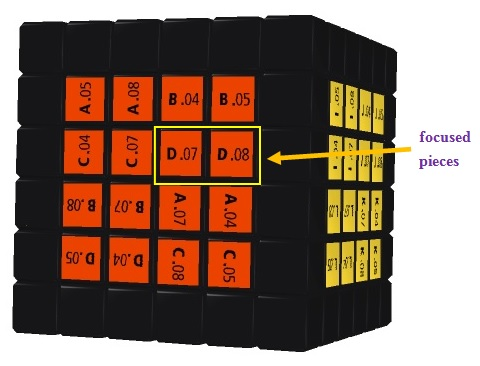While the method given above can accomplish the objective of Sub-step 2, it may also give rise to OLL parity errors. As a matter of fact, OLL parity errors arise when any hidden cross piece orbit has odd parity. You may wonder: why bother about cross pieces as there are no cross pieces on an even-order Supercube? But remember that even-order Supercubes can be seen as odd-order Supercubes with the core inner layer hidden, as shown in the following diagram:Thus, although the cross pieces are invisible on an even-order Supercube, they do exist in the sense that the relative parities of the cross piece orbits will determine the emergence or non-emergence of OLL parity errors. But how can you determine the relative parities of the invisible cross piece orbits? One way is to make use of the following fact which is applicable to odd-order and even-order Supercubes alike:

Fact 3: The relative parity of a cross piece orbit and the absolute parity of a non-core edge piece orbit that are located on the same non-core inner layer are equal to each other.

For example, to find the relative parity of the hidden cross piece orbit on the Supercube in the diagram above (there is only one such orbit in a 4th order Supercube), you can determine the absolute parity of the "01-04" orbit, because the hidden cross piece orbit and the "01-04" non-core edge piece orbit are located on the same non-core inner layer. So the question becomes: how to determine the absolute parity of an orbit? The basic principle for determining the absolute parity of a particular orbit is to trace where the pieces of that orbit have moved to and then calculate the parity of these moves. I show you how to do this by means of an example.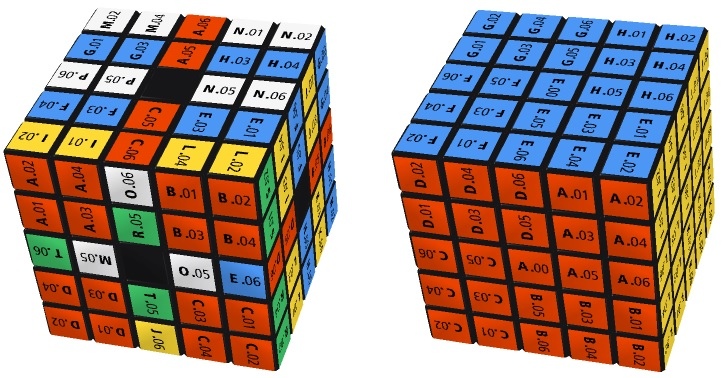The diagram on the left above shows a 5th order Supercube which has been scrambled. The core center pieces of this Supercube have been darkened to highlight the fact that for the purpose of determining the absolute parity of an orbit, it does not matter how the Supercube is oriented. Suppose you hope to determine the absolute parity of the "01-04" orbit. You first choose any piece in this orbit, say the "A.01" piece (strictly speaking, this should be called the "A.01-E.04" piece. But to simplify notation, here I write "A.01" instead), and trace where it has gone. By comparing the scrambled Supercube with the solved 5th order Supercube on the right above, you can see that the "A.01" piece has moved to where the "D.01" piece used to be. You can record this by writing (A.01, D.01 ...). This is called a cycle notation. To continue, you then trace where the "D.01" piece has gone. By comparing the diagrams again, you can see that the "D.01" piece has moved to where the "C.01" piece used to be. You then modify the previous cycle notation as (A.01, D.01, C.01 ...). Proceeding in this way, your modified cycle notation will be like (A.01, D.01, C.01, B.01, A.01, D.01, C.01, B.01, A.01 ....), endless. To avoid writing out endless cycles, you can just write this cycle as (A.01, D.01, C.01, B.01) by assuming the convention that this cycle notation means that the last piece in the cycle (i.e. "B.01") moves to where the first piece in the cycle (i.e. "A.01") used to be.

So far you have only dealt with 4 pieces and found that they form a cycle themselves. There are 20 more pieces in the "01-04" orbit. So you next choose an arbitrary piece that has not been dealt with, say the "E.01" piece, and trace where it has gone. By comparing the diagrams again, you can see that the "E.01" piece remains where it used to be. You record this by writing (E.01), i.e. a cycle containing only 1 piece and append this new cycle with the cycle written in the previous paragraph: (A.01, D.01, C.01, B.01)(E.01). You then choose another piece that has not been dealt with, say the "I.01" piece, and trace where it has gone. Since the "I.01" piece has moved to where the "F.01" piece used to be, you then modify your cycle notation as (A.01, D.01, C.01, B.01)(E.01)(I.01, F.01 ...). Proceeding in this way until you have traced all the 24 pieces in the "01-04" orbit, you will be able to write out a complete cycle notation for this orbit such as the following:

(A.01, D.01, C.01, B.01)(E.01)(I.01, F.01, P.01, S.01)(G.01)(H.01, K.01, Q.01, N.01)(J.01)(L.01)(M.01)(O.01)(R.01)(T.01)(U.01, X.01, W.01, V.01)

After obtaining the cycle notation for an orbit, you then do the following: for each cycle in the cycle notation, count the number of pieces it contains and subtract 1 from that number, and then add all the numbers. The parity of the sum is then the absolute parity of that orbit. With the cycle notation shown above, you calculate 3 + 0 + 3 + 0 + 3 + 0 + 0 + 0 + 0 + 0 + 0 + 3 = 12. Since 12 is even, the "01-04" orbit in the diagram above has even absolute parity.

I now return to the discussion of OLL parity errors. To avoid causing these errors, when doing Sub-step 1 of Step III, instead of checking the relative parities of the non-core center piece orbits, you should check the absolute parities of the non-core edge piece orbits. By virtue of Fact 3, these absolute parities are equal to the relative parities of the corresponding cross piece orbits. In case any of these parities is odd, you then apply the One Side Swapping Formula (15) to every non-core inner layer on the right half of the red face that contains a cross piece orbit with odd relative parity. For illustration, consider the following diagram:The diagram above shows two views of a 6th-order Supercube with the core inner layer hidden. For convenienece, I have labelled the two hidden cross pieces on the right half ot the red face as "M1" and "M2". Under the old solution method (Step III), the focused pieces on the red face are the "D.08" and "A.07" pieces. The alphabet lists for the "08" and "07" orbits are "ACBD" and "BDCA", respectively (to ensure even relative parity for the "07" point piece orbit, you should start writing the alphabet lists from the bottom right hand corner of the red face). Since "ACBD" has odd parity, under the old method you have to apply formula (15) to the inner layer with the "M1" cross piece. But this will in fact give rise to the OLL parity errors.

If you use the method introduced above, you will find that the absolute parity of the "02-06" orbit (equal to the relative parity of the M1 orbit) is even, while the absolute parity of the "01-09" orbit (equal to the relative parity of the M2 orbit) is odd. Thus, under the new method you should apply formula (15) to the inner layer with the "M2" cross piece. In this way, not only can you accomplish the objectives of Step III, but you will also avoid the OLL parity errors.

## PLL Parity Errors of Even-order Supercubes

The PLL parity errors are illustrated by the following diagram: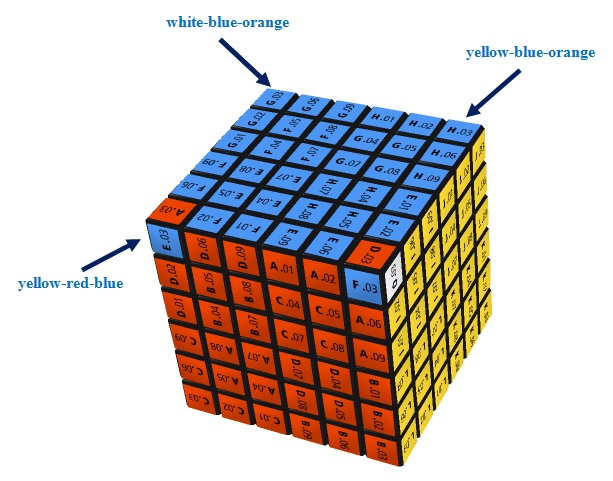The above figure shows a 6th-order Supercube. Among the 4 corner pieces on the top face of this Supercube, exactly two (i.e. the "yellow-blue-orange" and "white-blue-orange" corner pieces) are placed correctly. Generally speaking, we say that a PLL parity error occurs whenever there are exactly 2 corners placed correctly (not necessarily both oriented correctly) when you do Sub-step 6 of Step VI. Note that this type of errors will only arise in even-order Supercubes. In TUTORIAL ON SUPERCUBE SOLVING (3) (Solving Even-Order Supercubes), I provided a method for dealing with the aforesaid situation. This method has to make use of the Two Side Swapping Formula (16) and Two Side Flipping Formula (18). Moreover, you will also have to re-do Sub-step 5 after applying these two formulas.

I must confess that I do not know how to avoid the occurrence of PLL parity errors. But at least there is a method that can avoid re-doing Sub-step 5 and so can improve the solution method a bit. According to 7 Steps Solution Guide, when doing Sub-step 5 of Step VI, you first hold the cube with the red face in front and then rotate the top face so that the "red-blue" edge piece is facing forward. Instead of proceeding as instructed in the Solution Guide at this moment, you should calculate the absolute parity of the corner orbit. Note that all corner pieces comprise an orbit, and I have already introduced how to calculate the absolute parity of any orbit. After doing the calculation, you should then check whether the absolute parity of the corner piece orbit is compatible with the color arrangement of the top face according to the following diagram: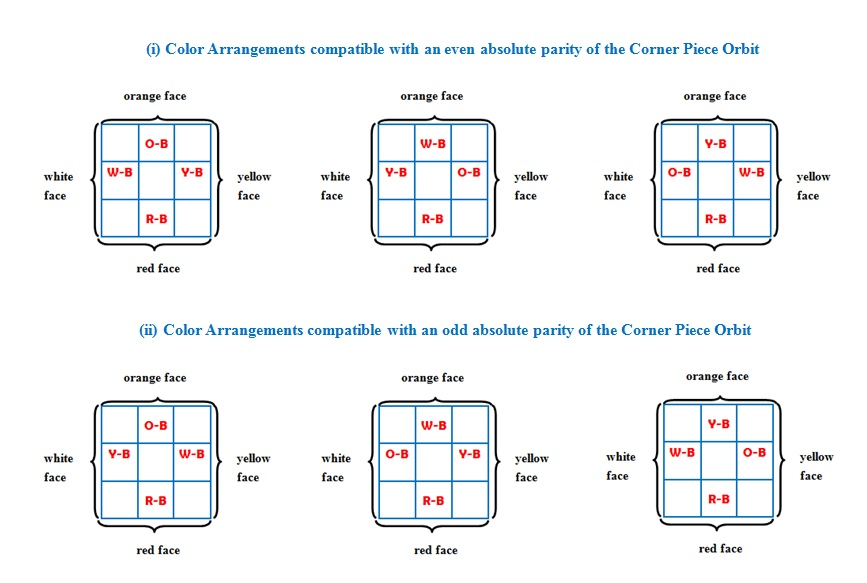The diagram above shows six possible color arrangements of the top face, where the letters R, O, Y and W represent the red, orange, yellow and white colors, respectively. If the absolute parity and the color arrangement are compatible with each other, there is no PLL parity errors and you can proceed with the remaining procedures in Sub-step 5 as instructed in the Solution Guide. If they are not compatible, then there is PLL parity error. You have to rotate the whole Supercube so that the white face faces forward and then apply formulas (16) and (18) before proceeding with the remaining procedures in Sub-step 5. For illustration, consider the diagram below: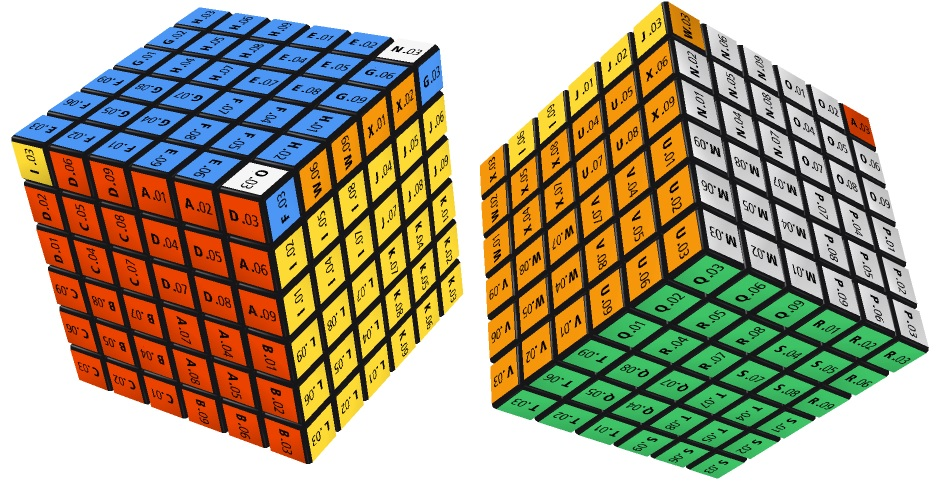In the 6th-order Supercube shown above, the colors of the edges on the yellow face, orange face and white face are O-B, Y-B and W-B, respectively. This color arrangement falls within type (ii) above, i.e. it is a color arrangement compatible with an odd absolute parity of the corner piece orbit. If you check the corner pieces in the diagram above, you will find that the corner piece orbit has even absolute parity. This means that there is PLL parity error. So you need to rotate the whole Supercube so that the white face faces forward and then apply formulas (16) and (18) (remember that when using these two formulas, you have to move the "02-06" and "01-09" edge pieces together). After doing these, the Supercube will become like this: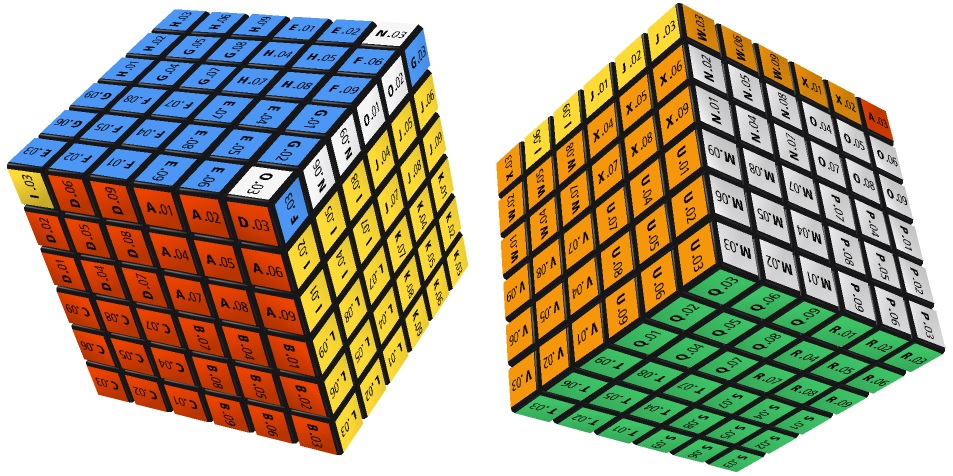You can now check that the colors of the edges on the yellow face, orange face and white face are W-B, Y-B and O-B, respectively. This color arrangement falls within type (i) above, Moreover, the absolute parity of the corner piece orbit remains to be even, which is now compatible with the color arrangement. You may then proceed with the remaining procedures in Sub-step 5 and need not worry that you have to re-do these steps because the PLL parity error has been rectified.

Go to Supercube Links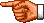Go toMy Personal Homepage (English Version)
Go toMy Personal Homepage (Chinese Version)